# Shunt Active Power Filter for Power Quality Improvement by using PI and Fuzzy Controller

A fuzzy logic-controlled shunt active power filter has been studied to improve the power quality by compensating harmonics and reactive power requirement of the nonlinear load.The simulation study of proportional integrator (PI) and fuzzy controlled DC bus voltage of three phases shunt active power filter (APF) to improve power quality by compensating harmonics by nonlinear load is proposed. The compensation process is based on sensing mains currents only, an approach different from conventional methods, which usually require harmonics or reactive volt-ampere values of the load. Pulse width modulation (PWM) signal generation is based on hysteresis control comparators and conventional PI controller to obtain the switching signals. In this paper a fuzzy logic-based controller is developed to replace conventional PI controller. Simulation works of the power circuit model, using SIMULINK under MATLAB software were carried out. The simulation results reveal that performance of fuzzy controller is better than PI controller.

The need for efficient use of electrical power has increased the areas of application of power electronics. Many such power electronic converters draw nonsinusoidal current and inject harmonics into the power distribution system. These harmonics lead to higher losses in the system, heating of equipment, voltage sags, swells and power factor reduction. Some of the harmonic producing loads are rectifiers, arc-furnaces, cycloconverters and adjustable speed drives. Many researches are working in this area in order to reduce the harmonic in power system in a desired level and to increase the power quality.

In early year, passive filter plays the major role in the contest of harmonic mitigation, but it has remarkable discrepancies. This was due to their low cost, simplicity and higher efficiency. But they are susceptible to series and parallel resonances with the supply and the load, and their filtering characteristics are influenced by the supply impedance. Tuned passive filters are also susceptible to load and line switching transients and they are always off-tuned. Hence active filtering became necessary.

With remarkable progress in speed and capacity of semiconductor switching devices such as GTO, Thyristor and IGBT, active filter consisting of voltage or current source pulse width modulation (PWM) inverters have been studied and put into practical use because they have the ability to overcome the mentioned disadvantages inherent in passive filters. Generally, PWM inverter can be divided into two main categories. It is current source or voltage source PWM inverter. The significance different between them is the energy storage element. Where, current source PWM inverter using reactor while voltage source PWM inverter using capacitor. Voltage PWM inverter is a preference because it is lower initial cost and more efficient compare to the other.

The effectiveness of active power filter depends on requirements, inverter type, configuration and the control strategy which has to be selected appropriately. Active filters can be configured mainly in four connections:
• Series connected active power filter.
• Shunt connected active power filter.
• Hybrid series and shunt active power filter.
• Hybrid series active power and passive filter.
Series connected active power filter is connected before the load in series with the mains using a matching transformer to eliminate the voltage harmonics and to balance and regulate the terminal voltage of the load. Also, it can be used to regulate negative sequence voltage at the load. So, the series active filter works as a controllable voltage source. Shunt connected active power filter is widely used to eliminate current harmonics, reactive power compensation and to balance the unbalance currents. It is mainly used at the load end because current harmonics are injected by nonlinear loads. It injects equal compensating currents opposite in phase to cancel harmonics and reactive components of the nonlinear load currents at the point of connection.

As resultant, a new approach to rating reduction in active filter has been proposed on the basis of the combination of active filter and passive filter. The passive filter suppresses harmonic current produced by the load and improves the power factor of the system. Whereas the active filter improves the filtering characteristics of passive filters and eliminates the voltage and current harmonic of the load. As a result, the proposed system can solve the problems inherent in using only the passive filter.

In active filter so many techniques for inverter control are available. Harmonic current detection is a well-known technique. Also, Instantaneous Reactive Power theory, Fast Fourier Transforms method (FFT), Analog notch filter (Low pass, High pass and Band pass filter) and Active and Reactive power calculation method is widely used for this purpose. In this paper notch filter method using band pass filter was used.

With SIMULINK, Simulation model of 3- phase Line circuit from Source to R-L load has been developed. Between source and load, Active Power filter is connected as shown in fig.1 such that it will feed harmonic component of current, so that the current wave form of source will be almost Sinusoidal. A non-linear load consists a diode bridge rectifier, is used to generate harmonics in system. The active power filter is supplied getting gatting signal either by PI controller or fuzzy controller. The performance of system is studied for varying load condition by using both controllers.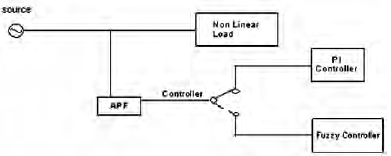Figure 1: Block Diagram of APF Controller

Conventional PI controller is very difficult to tuned (Kp, Ki parameters) under varying load condition. To improve performance APF, conventional PI controller is replaced by fuzzy controller. Fuzzy Logic Controllers (FLCs) have generated a good deal of interest in certain applications. The advantages of FLCs over the conventional controllers are:
• Does not need accurate mathematical model.
• Can work with imprecise inputs.
• Can handle nonlinearity, and
• More robust than conventional nonlinear controllers.

So far, FLCs in power electronics have been designed by trial and error. Power converters are inherently nonlinear. The causes of nonlinearity in the power converters include a variable structure within a single switching period, saturating inductances, voltage clamping, etc. With the advent of resonant converters, power converters are getting complicated, resulting in complex mathematical models. The FLC seems to be a viable controller for power electronic applications.

Shunt Active Power Filter
Shunt Active Power Filter (APF) is most important configuration and widely used in active filtering applications. A shunt APF consists of a controllable voltage or current source. The voltage source inverter (VSI) based shunt APF is by far the most common type used today, due to its well-known topology and straight forward installation procedure. Figure 2 shows the principle of shunt APF. Shunt APF acts as a current source, compensating the harmonic currents due to nonlinear loads. The receiver, which absorbs the whole or part of the disturbances generated by the said receiver If we denote ica, icb, icc the receiver absorbed currents, and isa, isb, isc, the desired power supply currents, then the active filter must provide currents ifa, ifb, ifc, given by,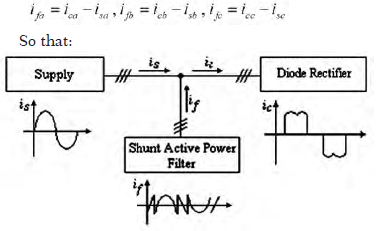Figure 2: Shunt active power filtering principle

1]Currents taken from the power supply are sinusoidal.
2 Fundamentals of these currents are in phase with the supply voltages.
The currents meet these two conditions simultaneously.
The shunt active power filter is intended to generate exactly the same harmonics contained in the polluting current ic but with opposite phase. The equivalent circuit of the whole power supply-active filter-diode rectifier is presented in Figure 3. It consists of a DC-bus capacitor (Cdc ), power electronic switches and interfacing inductors (Lf).

Figure 4 illustrates the active power filter control circuit synoptic diagram, where ‘PI’ is a Proportional- Integrator corrector.

A good and robustness control is a system which forces a signal to track closely its reference. A carrier based PWM control strategy is used for getting gatting signals for bridge circuit.Fig.4.Control circuit synoptic diagram

Reference current for calculating irefi (i = a, b, c) are proposed. In this paper notch filter circuit based on second order band pass filter used to remove fundamentals while leaving the harmonic components, as shown in Figure 5. A single notch filter with a bandwidth of 5Hz has good isolating characteristics. The filter can significantly reduce the output THD and can recover from a step change transient in 10 fundamental cycles. An active filter which uses a notch filter on each of the three phases to determine the compensating current can cope with unbalanced three-phase loads. Figure 5 shows the block diagram for an active power filter that uses a notch filter. The load current is filtered to leave the harmonics. The harmonic currents are subtracted from the load current by injecting into the power line with a 180-degree phase shift.Fig.5. Notch filter based on band-pass filters method.

Fuzzy Control Application
Fuzzy logic serves to represent uncertain and imprecise knowledge of the system, whereas fuzzy control allows taking a decision even if we can’t estimate inputs/ outputs only from uncertain predicates. Figure 6 shows the synoptic scheme of fuzzy controller, which possesses two inputs: the error (e), (e = iref – if) and its derivative (de), and one output: the command (cde). This three variables used as numerical variables from the real system. To convert numeric variables into linguistic variables, the following five fuzzy sets are chosen as: GN (grand positive), Z (zero), P (positive), N (negative) and GP (grand positive).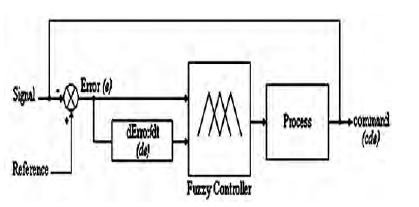Figure 6: Fuzzy controller synoptic diagram

Fuzzy control of the active power filter’s current
The main characteristics of the fuzzy control are:
• Three fuzzy sets for each input (e, de) with
Gaussian membership functions, as shown in fig.7.
• Five fuzzy sets for the output with triangular
membership functions, as shown in fig.7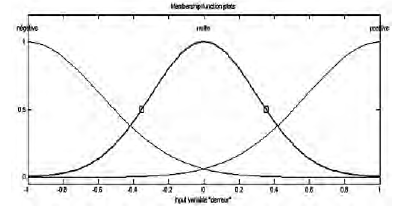Fig.7.Membership function plot for error (e), change
in error (de) and output (cde)

• Implications using the ‘minimum’ operator, inference mechanism based on fuzzy implication containing five fuzzy rules, for ‘minimum’ operator AND logic used. In AND logic, two input error (e) and change in error (de) compare with minimum operator and getting output in triangular membership function plot.
• The establishment of the fuzzy rules is based on the error (e) sign, variation and knowing that (e) is increasing if its derivative (de) is positive, constant if (de) is equal to zero, decreasing if (de) is negative, positive if (iref > if), zero if (iref = if), and negative if (iref < if), fuzzy rules are summarized as following:
1. If (e) is zero (Z), then (cde) is zero (Z).
2. If (e) is positive (P), then (cde) is grand positive (GP).
3. If (e) is negative (N), then (cde) is grand negative (GN).
4. If (e) is zero (Z) and (de) is positive (P), then (cde) is negative (N).
5. If (e) is zero (Z) and (de) is negative (N), then (cde) is positive (P).
The evaluation of the five rules have an AND logical operator, the DOF (degree of fulfilment) of each rule selects only the minimum value.
• Defuzzification using the ‘centroid’ method.

Simulation
The simulation programs developed in MATLAB Simulink environment, can visualize in a given time interval currents and voltages evolution in different branches of APF circuit. THE PARAMETERS SELECTED FOR SIMULATION STUDIES ARE: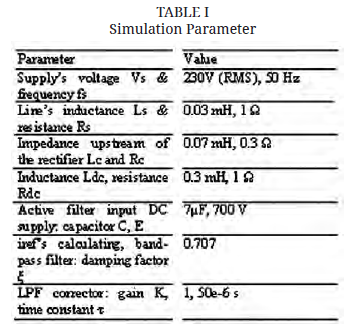Figures 8 and 10 show waveforms of load current, source current and filter current respectively. The instant filter is switched on source current becomes sinusoidal from stepped wave shape. Filter switched on at 0.04 second. Figure 10 shows source current using PI controller.Time
Fig.8.Waveforms of load current, source current and filter
current APF with Fuzzy Controller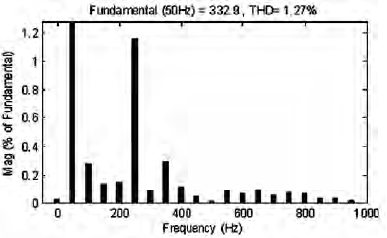Fig.9.Source current spectrum with filter at Fuzzy Controller.Figure 10: Waveforms of load current, source current and
filter current APF with PI Controller.Figure 11: Source current spectrum with filter at PI controller.

Discussion
The controllers for PI and FLC were designed to regulate the output voltage under supply voltage disturbances and load disturbances. At first, let see in Figures 9 and 11 the source current waveform and its harmonic spectrum before the application of classical shunt active power filtering. Important distortions are noticed in these figures with a THD of 20.51%.

After the application of shunt active power filtering based on PI controller, the curves mentioned in Figures 10 and 11 are obtained presenting the supply’s current waveform and its harmonic spectrum in phase a ( ). It’s clear that distortion was attenuated at a satisfying level seen that the THD decreased to 1.70%. An excellent performance of the APF system when using a fuzzy logic controller has been observed, its THD is reduced to 1.27 per cent Figures 8 and 9 show the effectiveness of the control strategy which forced the APF current to follow its reference.

Above result shows at load R=1Ω. After than varied load condition, and taken load resistance at R=5 Ω. The harmonic analysis shown in Table 2. From this observed that, the performance of fuzzy logic better than PI controller.Conclusion
The study of fuzzy logic control, PI control suggests that FLC performs satisfactorily in regulating the output during external disturbances. The transient overshoot in FLC is negligible compared to PI response.

A fuzzy logic-controlled shunt active power filter has been studied to improve the power quality by compensating harmonics and reactive power requirement of the nonlinear load. The performance of a fuzzy logic-controlled shunt active power filter has been studied and compared with the conventional PI controller. The fuzzy controller has better transient response compared to a conventional PI controller, The THD of the source current after compensations as well below 5%, the harmonic limit imposed by the IEEE-519 standard. An excellent performance of the APF system when using a fuzzy logic controller has been observed.

It is intended to extend this study to the hybrid structure of series and shunt active power filter and application of the neural networks. Hybrid power filter which cannot reduce the source current harmonics but able to improve the source voltage waveforms in case of heavy load conditions. Also, APF simulate with neurofuzzy controller.Ms. Chandani Parmar,